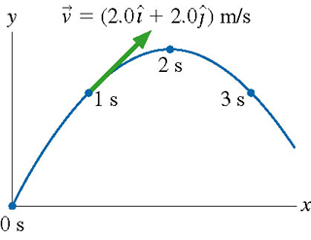# Problem: A physics student on Planet Exidor throws a ball, and it follows the parabolic trajectory shown in the figure . The balls position is shown at 1s intervals until exttip{t}{t} =3s. At exttip{t}{t} =1s, the balls velocity is exttip{vec{v}}{v_vec} =( 2.0+ 2.0 )/s.Determine the balls velocity at exttip{t}{t} =0.Determine the balls velocity at exttip{t}{t} =2s.Determine the balls velocity at exttip{t}{t} =3s.What is the value of exttip{g}{g} on Planet Exidor?What was the balls launch angle?

⚠️Our tutors found the solution shown to be helpful for the problem you're searching for. We don't have the exact solution yet.

###### Problem Details

A physics student on Planet Exidor throws a ball, and it follows the parabolic trajectory shown in the figure. The balls position is shown at 1s intervals until =3s. At =1s, the balls velocity is =( 2.0+ 2.0 )/s.

Determine the balls velocity at =0.

Determine the balls velocity at =2s.

Determine the balls velocity at =3s.

What is the value of on Planet Exidor?

What was the balls launch angle?